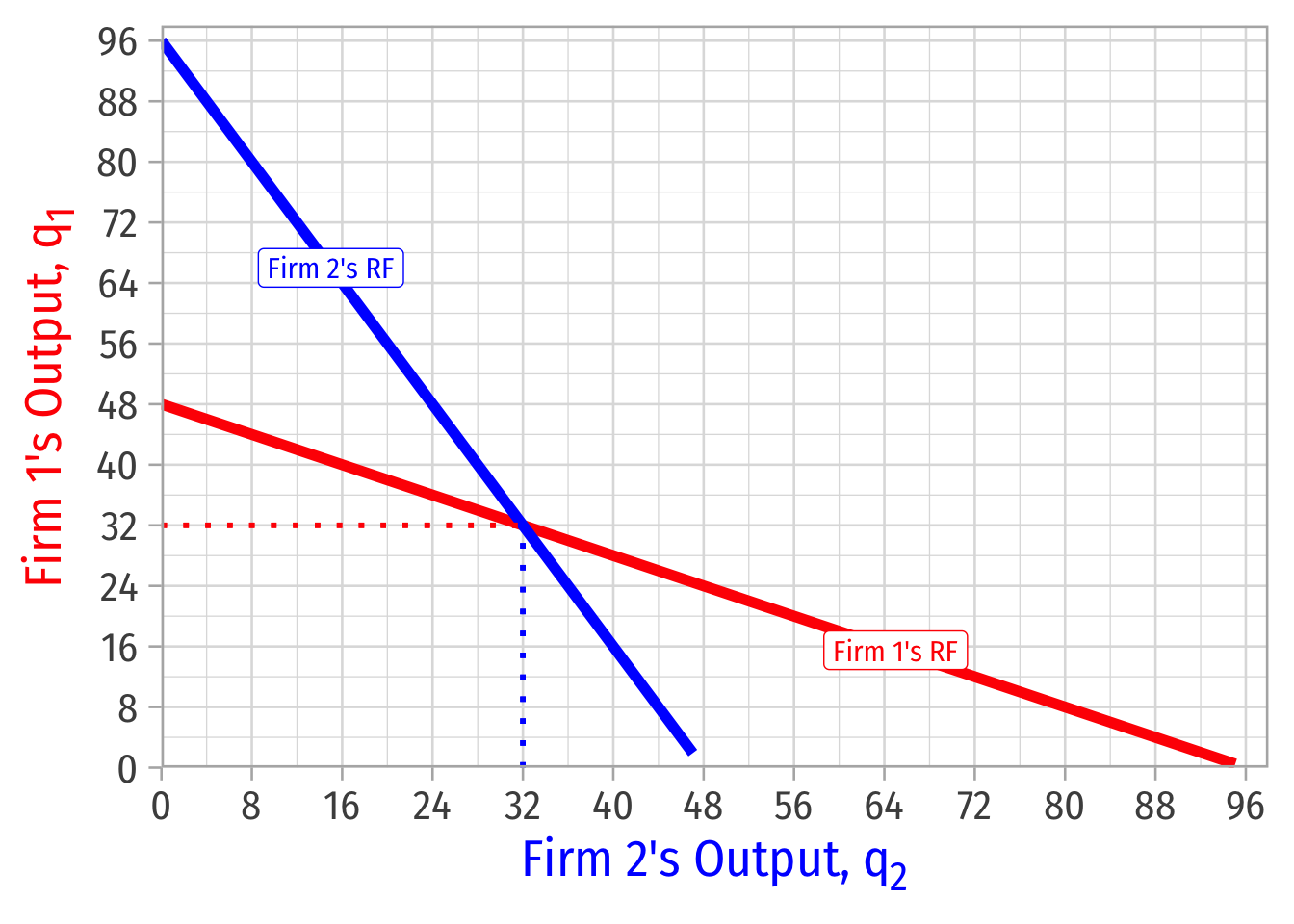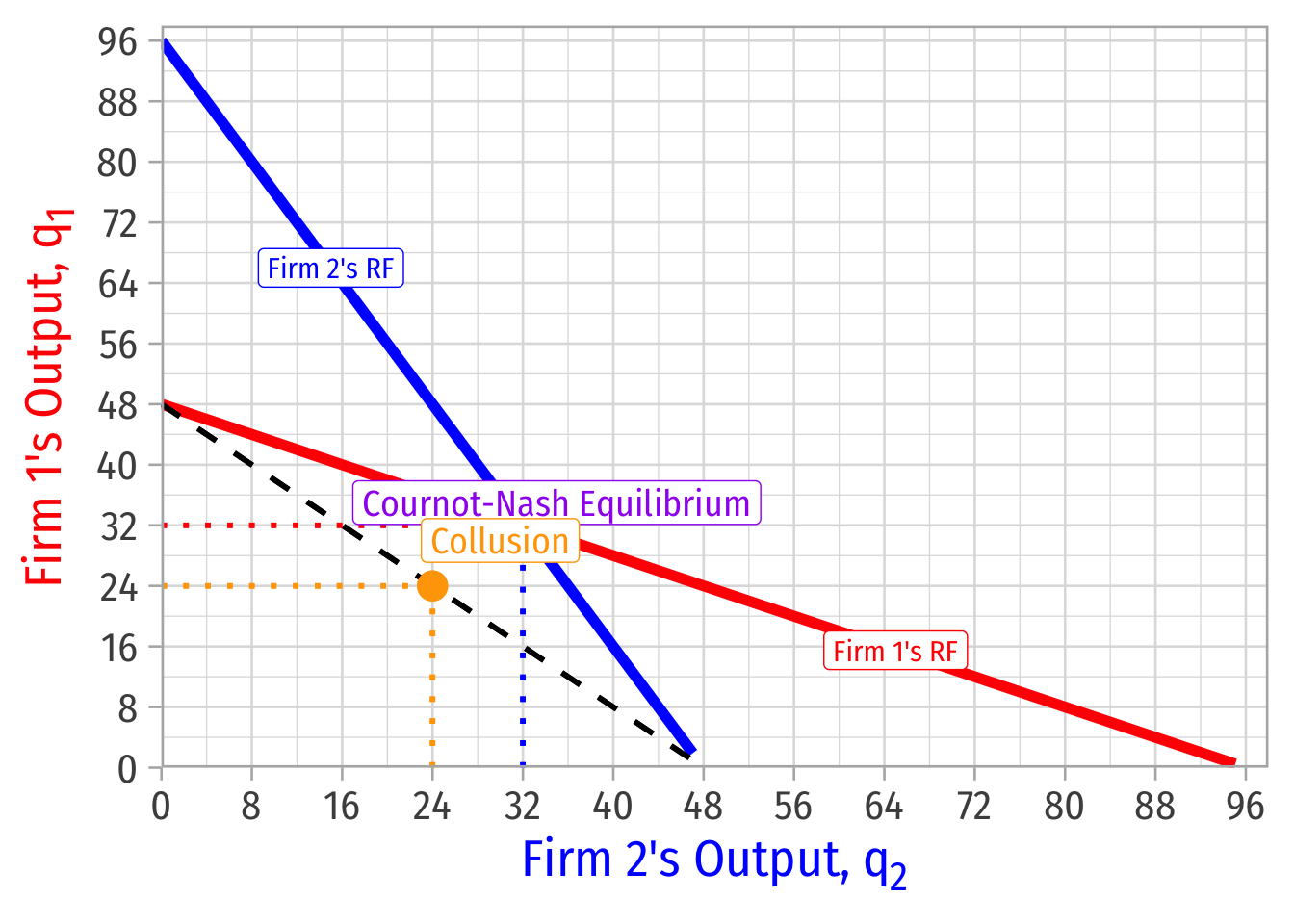Suppose Firm 1 and Firm 2 have a constant $$MC=AC=8$$. The market (inverse) demand curve is given by:

\begin{aligned} P&=200-2Q\\ Q&=q_1+q_2\\ \end{aligned}

1. Find the Cournot-Nash equilibrium output and profit for each firm if they compete on quantity.

Break demand apart into both firms’ output.

\begin{align*} P&=200-2Q\\ P&=200-2(q_{1}+q_{2})\\ P&=200-2q_{1}-2q_2\\ \end{align*}

Solving for Firm 1, recalling that MR is twice the slope of the inverse demand curve: $MR_{1}=200-4q_{1}-2q_2$

Firm 1 maximizes profit at $$q^*$$ where $$MR=MC$$:

\begin{align*} MR_{1}&=MC && \text{Profit-max condition} \\ 200-4q_{1}-2q_2&=8 && \text{Plugging in}\\ 192-4q_1-2q_2&=0 && \text{Subtracting 8 from both sides}\\ 192-2q_2&=4q_1 && \text{Adding }4q_{1} \text{ to both sides}\\ 48-0.5q_1&=q_1^* && \text{Dividing both sides by 4}\\ \end{align*}

Since Firm 2 is identical, its $$q^*$$ is: $q_2^*=48-0.5q_{1}$

Find Nash equilibrium algebraically by plugging in one firm’s reaction curve into the other’s

\begin{align*} q_{1}&=48-0.5q_2 && \text{Firm 1's reaction function}\\ q_{1}&=48-0.5(\textcolor{blue}{48-0.5q_{1}}) && \text{Plugging in Firm 2's reaction function}\\ q_{1}&=48-24+0.25q_{1} && \text{Distributing the }-0.5\\ q_{1}&=24+0.25q_{1} && \text{Subtracting}\\ 0.75q_{1}&=24 && \text{Subtracting }0.25q_{1} \text{ to both sides}\\ q_{1}&=32 && \text{Dividing by 0.75}\\ \end{align*}

Symmetrically, $$q_{2}=32$$Both firms produce 32:

\begin{align*} P&=200-2(32)-2(32)\\ P&=\72\\ \end{align*}

We can find the profit for each firm:

\begin{align*} \pi_{1}&=q_{1}(P-c)\\ \pi_{1}&=32(72-8)\\ \pi_{1}&=\2,048\\ \end{align*}

1. Find the output and profit for each firm if the two were to collude.

Suppose now both firms collude and act like a single monopolist, who sets:

\begin{align*} MR&=MC && \text{Profit-max condition}\\ 200-4Q&=8 && \text{Plugging in}\\ 192-4Q&=0 && \text{Subtracting 8 from both sides}\\ 192&=4Q && \text{Adding }4Q \text{ to both sides}\\ 48&=Q && \text{Dividing both sides by 4}\\ \end{align*}

So each firm will produce 24.The monopoly price will then be \begin{align*} P&=200-2Q\\ P&=200-2(48)\\ P&=\104\\ \end{align*}

Total profit will then be: \begin{align*} \Pi&=Q(P-c)\\ \Pi&=48(104-8)\\ \Pi&=\4,608\\ \end{align*}

with \$2,304 going to each firm.

1. Find the Nash equilibrium price each firm charges if they compete on price.

We know the Bertrand-Nash equilibrium is the perfectly competitive one, i.e. where $$p=MC$$.

\begin{align*} P&=MC\\ 200-2Q&=8\\ 192-2Q&=0\\ 192&=2Q\\ 96&=Q\\ \end{align*}

Each firm produces 48.

1. Find the industry price, output, and profits under this equilibrium (in part 3).

The price should be marginal cost, and profits should be zero, but we can confirm:

\begin{align*} P&=200-2Q\\ P&=200-2(96)\\ P&=\8\\ \end{align*}

Total profit will then be: \begin{align*} \Pi&=Q(P-c)\\ \Pi&=96(8-8)\\ \Pi&=\0\\ \end{align*}

Comparing the different equilibria: